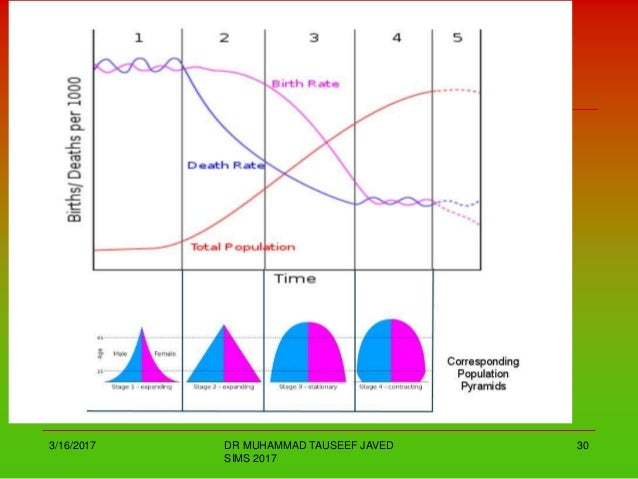# Population Statistics, Graphs and Tables of Pakistan

This post illustrates and interprets different tables, graphs, and statistics of the Population of Pakistan. It also explains the formula used in the calculation of birth, death, and birth rates, and related concepts.

Contents

### How is the crude birth rate calculated?

The crude birth rate is calculated by dividing the number of births by1000 and multiplied by 100. It may simply be defined as the Number of births per 1000 people multiplied by 100.

The formula can be put as follows:

No. of births/1000 x 100= Crude birth rate

### How is the crude death rate calculated?

The crude death rate is calculated by dividing the number of deaths by 1000 and multiplied by 100. It can be simply be defined as the Number of births per 1000 people multiplied by 100.

The formula can be put as follows:

#### No. of death/1000 x 100= Crude birth rate

Death and birth rate of Pakistan over the years

Survey Period

Birth rate per 1000 The crude death rate per 1000

1984 – 1988

42.7 10.9

1989 – 1994

39.5

10.1

1996

35.5

8.7

1998

32.7

9.1

2004

28.0

8.10

2009

25

7.7

### Interpretation of the table

The statistics of the population of Pakistan given in the above table illustrate that has been a consistent decrease in the crude birth rate in Pakistan. According to the table, the crude death rate also decreased from 1984 till 1996 but it was increased in 1998. It has consistently decreased since 1998 till date.  Thus, it can be concluded that birth and death rates in Pakistan have decreased consistently over the years.

### Percentage of population growth from 1951-2008

 Year Annual growth rate 1951 1.7 1961 2.4 1971 3.69 1981 3.06 1983 2.99 1987 2.82 1988 2.77 1989 2.73 1993 2.56 1994-95 2.51 1995-96 2.43 1996-97 2.38 1998 2.34 1999 2.29 2000 2.24 2001 2.22 2002 2.16 2003 1.94 2004 1.90 2008 1.80 Economic Survey of Pakistan 2007 – 08

### Interpretation of the table

The growth rate of Pakistan increased consistently in the first two decades from 1951 to 1971. After 1983 there has been a consistent decrease in the natural increase rate of the population of Pakistan as illustrated in the demographic transition model of Pakistan.

### Demographic Transition Model of PakistanPic Courtesy: Dr. Muhammad Tauseef Javed SIMS 2017

### Noor Akber

I am a social activist and educationist. I write on politics, culture, education and economy.